Impact Factor 3.515 | CiteScore 2.7
More on impact ›

# Frontiers in Materials## ORIGINAL RESEARCH article

Front. Mater., 17 March 2021 | https://doi.org/10.3389/fmats.2020.589701

# Exploring Metamaterials’ Structures Through the Relaxed Micromorphic Model: Switching an Acoustic Screen Into an Acoustic AbsorberGianluca Rizzi1,Manuel Collet2,Félix Demore2,Bernhard Eidel3,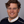Patrizio Neff4* andAngela Madeo1
• 1GEOMAS, INSA-Lyon, Université de Lyon, Villeurbanne Cedex, France
• 2École Centrale de Lyon, Écully, France
• 3Heisenberg-Group, Institute of Mechanics, Department Mechanical Engineering, University Siegen, Siegen, Germany
• 4Head of Chair for Nonlinear Analysis and Modelling, Fakultät für Mathematik, Universität Duisburg-Essen, Essen, Germany

While the design of always new metamaterials with exotic static and dynamic properties is attracting deep attention in the last decades, little effort is made to explore their interactions with other materials. This prevents the conception of (meta-)structures that can enhance metamaterials’ unusual behaviors and that can be employed in real engineering applications. In this paper, we give a first answer to this challenging problem by showing that the relaxed micromorphic model with zero static characteristic length can be usefully applied to describe the refractive properties of simple meta-structures for extended frequency ranges and for any direction of propagation of the incident wave. Thanks to the simplified model’s structure, we are able to efficiently explore different configurations and to show that a given meta-structure can drastically change its overall refractive behavior when varying the elastic properties of specific meta-structural elements. In some cases, changing the stiffness of a homogeneous material which is in contact with a metamaterial’s slab, reverses the structure’s refractive behavior by switching it from an acoustic screen (total reflection) into an acoustic absorber (total transmission). The present paper clearly indicates that, while the study and enhancement of the intrinsic metamaterials’ properties is certainly of great importance, it is even more challenging to enable the conception of meta-structures that can eventually boost the use of metamaterials in real-case applications.

## 1 Introduction

The last decade has seen the birth of a true research outburst on so-called mechanical metamaterials which are able to show exotic mechanical properties both in the static and dynamic regime. Theoretical, experimental and numerical studies have flourished all around the world providing new insights in the domain of material properties manipulation which, only few years ago, was thought far from being prone to possible ground-breaking evolutions. We are today assisting to the conception and subsequent realization of new materials which, simply thanks to their internal architecture, go beyond the materials’ mechanical properties that we are used to know and which, for this reason, are called mechanical metamaterials. Already in the late 1980s, it was proven that some foams with special internal architecture can give rise to “negative Poisson” effects, i.e., they fatten when stretched, contrarily to what happens to the great majority of known materials which experience a reduction in the cross-section when submitted to tensile loads (Lakes, 1987). More recently, the frontiers of metamaterials’ conception are rapidly moving forward, giving rise to the manufacturing of always new metamaterials with more and more impressive properties. It is thus possible today to see 3D-printed pyramids connected by hinges giving rise to a block that is hard like a brick on one side but soft like a sponge on the other (Bilal et al., 2017), “unfeelability” cloaks hiding to the touch objects put below them (Milton and Cherkaev, 1995; Kadic et al., 2014), plastic cubes made out of smaller plastic cubes giving rise to bizarre deformations when squeezed (Coulais et al., 2016), or even metamaterials exploiting microstructural instabilities to change their mechanical response depending on the level of externally applied load (Kochmann and Bertoldi, 2017). When considering the dynamical behavior of mechanical metamaterials, things become, if possible, even more impressive, given the unusual responses that such metamaterials can provide when coming in contact with elastic waves (Deymier, 2013; Hussein et al., 2014; Barchiesi et al., 2019). It is today possible to find researchers working on metamaterials exhibiting band-gaps (Liu et al., 2000; Wang et al., 2014; Zhu et al., 2015; Bilal et al., 2018; El Sherbiny and Placidi, 2018; Celli et al., 2019; Goh and Kallivokas, 2019; Koutsianitis et al., 2019), cloaking (Norris et al., 2014; Bückmann et al., 2015; Misseroni et al., 2016; Misseroni et al., 2019), focusing (Guenneau et al., 2007; Cummer et al., 2016), channelling (Kaina et al., 2017; Tallarico et al., 2017; Wang et al., 2018; Bordiga et al., 2019; Miniaci et al., 2019), negative refraction (Zhu et al., 2015; Srivastava, 2016; Willis, 2016; Bordiga et al., 2019; Lustig et al., 2019; Morini et al., 2019), etc., as soon as they interact with mechanical waves.

Notwithstanding the massive research efforts deployed to unveil new metamaterials’ performances, researchers have just begun to understand the underlying mechanisms, so that “many designs so far have relied on luck and intuition” (Bertoldi et al., 2017).

In order to provide deeper theoretical insight into the mechanisms which allow to tailor metamaterials’ mechanical properties, so-called homogenization techniques have been developed which provide rigorous predictions of the macroscopic metamaterials’ mechanical behavior, when knowing the properties of the base materials and their spatial distribution. Homogenization techniques have proven their effectiveness for the description of metamaterials’ bulk behavior in the static and quasi-static regime (Hashin and Shtrikman, 1963; Hill, 1963; Willis, 1977; Sánchez-Palencia, 1980; Suquet, 1985; Allaire, 1992; Pideri and Seppecher, 1997; Miehe et al., 1999; Bouchitté and Bellieud, 2002; Milton, 2002; Camar-Eddine and Seppecher, 2003; Geers et al., 2010; Bensoussan et al., 2011) as well as, more recently, in the dynamic regime (Chen and Fish, 2001; Andrianov et al., 2008; Willis, 2009, 2011, 2012; Craster et al., 2010; Bacigalupo and Gambarotta, 2014; Boutin et al., 2014; Srivastava and Nemat-Nasser, 2014; Hu and Oskay, 2017; Srivastava and Willis, 2017; Sridhar et al., 2018).

While homogenization methods are effective to describe bulk metamaterials’ behaviors, they are intrinsically unsuitable to deal with metamaterials of finite size, because the ‘average operations’, on which they are built, make strong use of projection functions (e.g., Bloch-Floquet ones) that are defined in unbounded function spaces (Sridhar et al., 2016; Srivastava and Willis, 2017).

As a result of this gap, the response of finite-size metamaterials’ structures is today mostly explored via direct Finite Element (FEM) simulations that implement all the details of the involved microstructures (e.g., (Krushynska et al., 2017)). Despite the precise propagation patterns that these direct numerical simulations can provide, they suffer from unsustainable computational costs. Therefore, it is impossible today to explore large-scale meta-structures combining metamaterials and classical-materials bricks of different type, size and shape.

A first answer to this problem has been given by the introduction of the so-called relaxed micromorphic model without curvature contribution that has recently proven its effectiveness for the description of the mechanical behavior of a specific finite-size band-gap metamaterial with tetragonal symmetry (d’Agostino et al., 2020).

When compared to other techniques, this micromorphic approach shows its advantages at the considered macroscopic scale. In particular, with respect to the direct finite element implementation, it allows a rapid calculation (few hours vs. some weeks for the FEM simulation) of the metamaterials slab’s refractive properties for all angles of incidence and considered frequency ranges. With respect to dynamical and high-frequency homogenization, the presented approach allows to effectively deal with the finite-size of the metamaterial’s slab thanks to the introduction of well posed interface conditions guaranteeing the uniqueness of the searched solution.

Finally, other enriched continuum models such as couple stress or strain gradient models cannot describe band-gap metamaterials because they feature the same kinematics of a classical Cauchy continuum (only the displacement field). This implies that they can at best describe some dispersion in the acoustic curves, but cannot reproduce higher frequency optic modes.

In the present paper, we want to move beyond these first encouraging results and show how the relaxed micromorphic model without curvature contribution can be used to characterize many other tetragonal band-gap metamaterials that can be used for acoustic applications. To this aim, we will apply the inverse fitting procedure presented in (Neff et al., 2020; d’Agostino et al., 2020) to different metamaterials thus providing their mechanical description via the relaxed micromorphic model without curvature contribution.1 We will then explore how the behavior of these metamaterials can be profoundly changed by simply coupling them to classical homogeneous materials, realizing what we will call meta-structures, which can have very different mechanical properties when compared to those of the original metamaterial. The exploration of these new meta-structures is made possible thanks to the simplified structure of the relaxed micromorphic model without curvature contribution that allows quick computations of different structures obtained by embedding the selected metamaterial in different homogeneous materials. We show how the simple fact of changing the elastic properties of the external homogeneous material allows us to switch the structure’s behavior from total reflection to total transmission and vice-versa. It is clear that this new possibility of exploring different combinations of finite-size metamaterials and classical-materials opens concrete perspectives for the true employment of metamaterials in engineering design. Indeed, metamaterials’ reflection and transmission properties have been analyzed so far, always referring to the particular arrangement of their internal architecture so as to modify the metamaterial’s reflection/transmission behavior when the metamaterial is embedded, e.g., in air. Although such approaches can lead to the design of tunable interfaces allowing total transmission or total reflection depending on the topological microstructure’s properties (Zhou and Kriegsmann, 2007; Zhao et al., 2018; Park and Lee, 2019), they are not suitable to explore the behavior of the same metamaterials when they are used as building blocks of more complex structures that also contain other metamaterials and/or classical-materials elements, especially when these structures are widely extended in space.

As a matter of fact, these metamaterials that are conceived to act as perfect screens or perfect absorbers, could drastically change their response when embedded in homogeneous media or combined to other metamaterials. Little attention is given today to the need of exploring the response of “combined” classical-materials/metamaterials structures and this is preventing us from designing realistic meta-structures that control elastic waves and recover energy. Many authors have recognized the interest of studying the refractive behavior of metamaterials when combined to homogeneous materials to enhance unorthodox responses such as wave filtering (Bigoni and Movchan, 2002) or negative refraction (Brun et al., 2010; Willis, 2016). In a similar spirit, we present in this paper a simple meta-structure composed of a tetragonal metamaterial embedded in a homogeneous medium and we explore its wave-filtering response. The generality of our approach would allow us to study the response of the other structures cited so far (Willis, 1977, Willis, 2009; Bigoni and Movchan, 2002; Brun et al., 2010; Willis, 2016; Misseroni et al., 2019) and to highlight how the structure’s response could be modified when modifying simple parameters like the outer medium elasticity or the metamaterial’s characteristic size.

In this paper we show how the simple fact of assembling a finite-size metamaterial together with blocks of classical-materials can dramatically change the response of the metamaterial itself in such a way that the same structure may act as a complete absorber (total transmission) or a complete screen (total reflection). This switch of the structure’s dynamical properties is obtained by keeping the same geometry and the same metamaterial, while changing the homogeneous material in which the metamaterial itself is embedded. These results have been made possible thanks to the use of the relaxed micromorphic model without curvature contribution that, drastically reducing the computational time of the associated numerical simulations, could open the effective exploration of these new meta-structures. We clearly show that, while the interest of studying the intrinsic metamaterial’s properties by engineering its microstructure is certainly of great importance, it is even more important to unveil the effects of its interactions with other finite-size metamaterials and classical-materials bricks. It is indeed based on these interactions that it is possible to unfold new meta-structures which can further enhance the properties of the base metamaterials thus opening the way to realistic applications.

### 1.1 Notation

We recall here the notation that we will use throughout the paper. Let $ℝ3×3$ be the set of all real $3×3$ second order tensors which we denote by capital letters. A simple and a double contraction between tensors of any suitable order is denoted by $⋅$ and $:$ respectively, while the scalar product of tensors of suitable order is denoted by $〈⋅,⋅〉$.2 The Einstein sum convention is implied throughout this text unless otherwise specified. The standard Euclidean scalar product on $ℝ3×3$ is given by $〈X,Y〉=tr(X⋅YT)$ and consequently the Frobenius tensor norm is $X2=〈X,X〉$. The identity tensor on $ℝ3×3$ will be denoted by 1; then, $tr(X)=〈X,1〉$. We denote by $BL$ a bounded domain in $ℝ3$, by $δBL$ its regular boundary and by $Σ$ any material surface embedded in $BL$. The outward unit normal to $δBL$ will be denoted by ν as will the outward unit normal to a surface $Σ$ embedded in $BL$. Given a field a defined on the surface $Σ$, we define the jump of a through the surface $Σ$ as:

$〚a〛=a+−a−,witha−:=limx∈BL−∖Σx→Σa,anda+:=limx∈BL+∖Σx→Σa,(1)$

where $BL−,BL+$ are the two subdomains which result from splitting $BL$ by the surface $Σ$.

Classical gradient $∇$ and divergence Div operators are used throughout the paper.3 The subscript $,j$ indicates derivation with respect to the j−th component of the space variable, while the subscript $,t$ denotes derivation with respect to time.4

Given a time interval $[0,t0]$, the classical macroscopic displacement field is denoted by $u(x,t)∈ℝ3$, with $x∈BL, t∈[0,t0]$. In the framework of enriched continuum models of the micromorphic type, extra degrees of freedom are added through the introduction of the micro-distortion tensor P denoted by $P(x,t)∈ℝ3×3$, with $x∈BL, t∈[0,t0]$. This tensor can be related to micro-deformation mechanisms that take place at the scale of the unit cell and can account for dilation/compression-, shear- and rotation-like vibration modes, when different frequency levels are considered.

## 2 Equilibrium Equations, Constitutive Relations, and Energy Flux

### 2.1 Isotropic Cauchy Continuum

The equilibrium equations for the Cauchy continuum are

$ρ u,tt=Div[σ],(2)$

where σ is the Cauchy stress tensor. In the isotropic case, it takes the constitutive form $σ=2μ sym∇u+λ tr(sym∇u)1$, where λ and μ are the Lamé parameters and $sym∇u$ is the strain tensor.

When dissipative phenomena can be neglected, the following flux equation must hold:

$E,t+DivH=0,(3)$

where E is the total energy of the system and H is the energy flux vector, whose explicit expression is given by (see e.g., (Aivaliotis et al., 2018) for a detailed derivation)

$H=−σ⋅u,t .(4)$

### 2.2 Relaxed Micromorphic Continuum With Zero Static Characteristic Length

The equilibrium equations are obtained by looking for stationary points of the following action functional:

$A=∫​0t0∫BL(J−W)dXdt(5)$

where J is the kinetic energy density and W is the strain energy density of the considered micromorphic continuum.

In particular, the expression of the kinetic energy density takes the form (d’Agostino et al., 2020; Romano et al., 2016; Madeo et al., 2018a, Madeo et al., 2018b):5

where u is the macroscopic displacement field, $P∈ℝ3×3$ is the non-symmetric micro-distortion tensor, ρ is the macroscopic apparent density, and , $Jc$, $Te$, $Tc$ are 4th order micro-inertia tensors whose form will be specified in the following subsection.

The relaxed micromorphic continuum contains curvature terms connected to $CurlP$. Here, we use the relaxed micromorphic continuum without curvature contribution. The expression of the strain energy density without curvature contribution ($μ Lc22CurlP2=0$, $Lc=0$) is (d’Agostino et al., 2020; Romano et al., 2016; Madeo et al., 2018a, Madeo et al., 2018b):

where $ℂe$, $ℂmicro$, and $ℂc$ are 4th order tensors whose characteristic will be given in Section 2.3.

The minimization of the Action functional, Eq. 5, while using Eqs. 6, 7 provides the following equilibrium equations

where

The flux equation for the relaxed micromorphic continuum is formally the same as Eq. 3, but H has now the following expression (see (Aivaliotis et al., 2019b) for more details):

$H=−(σ˜+σ^,tt)T⋅u,t .(10)$

### 2.3 Particularization of the Relaxed Micromorphic Model to Plane Strain and Tetragonal Symmetry

We now focus on finding solutions in a plane strain framework. This means that we constrain the displacement field u and the micro-distortion P to depend only on the first two components $x1$ and $x2$ of the space variable x:

$u(x1,x2)=(u1(x1,x2)u2(x1,x2)0) , P(x1,x2)=(P11(x1,x2)P12(x1,x2)0P21(x1,x2)P22(x1,x2)0000) .(11)$

Given the metamaterials targeted in this paper (see Section 4), we particularize the equilibrium equations to the tetragonal case. This means that the elastic and micro inertia tensors appearing in Eqs. (6), (7) can be represented in the Voigt form as:

where only the coefficients involved in a plane strain problem are reported (the dots represent components acting on out-of plane variables and are not specified here).6

In the definition (13) of the micro-inertia tensors appearing in the kinetic energy (6), it is underlined the fact that they introduce dynamic internal lengths that can be directly related to the dispersion behavior of the metamaterial at very small (in the limit vanishing) wavenumbers (, $Jc$), as well as at very large (in the limit infinite) wavenumbers ($Te$, $Tc$).

## 3 Boundary Conditions for a Finite-Size Relaxed Micromorphic Slab Embedded Between Two Cauchy Half-Spaces

Two half-spaces made up of a homogeneous Cauchy material are separated by a micromorphic slab of finite width h. The three materials are in perfect contact with each other: the material on the top of the first interface is a classical linear elastic isotropic Cauchy medium, the material in the middle is an anisotropic relaxed micromorphic medium, while the material on the bottom of the second interface is again a classical isotropic Cauchy medium (see Figure 1).

FIGURE 1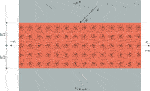FIGURE 1. Schematic representation of a wave with wavenumber k hitting at angle θ a relaxed micromorphic slab of thickness h embedded between two isotropic Cauchy media.

As it can be seen in (Madeo et al., 2016; Aivaliotis et al., 2018, Aivaliotis et al., 2019a) there are two boundary conditions which can be imposed at a Cauchy/relaxed-micromorphic interface if the static characteristic length ($Lc$) is zero (our case here): the continuity of displacement and continuity of generalized traction.

In the considered 2D case, there are then eight sets of scalar conditions, four on each interface. The finite slab has width h and we assume that the two interfaces are positioned at $x1=−h/2$ and $x1=h/2$, respectively (see Figure 1). The continuity of displacement conditions to be satisfied at the two interfaces of the slab are:

$uc−=us,onx1=−h2,us=uc+,onx1=h2,(14)$

where $uc−$ and $uc+$ are the displacement of the “minus” ($x1<0$) and “plus” ($x1>0$) Cauchy half-space, respectively. As for the continuity of generalized traction, we have:

where $tc±=σ±⋅ν±$ are classical Cauchy tractions, $ts=(σ˜+σ^,tt)⋅ν$ is the generalized traction in the relaxed micromorphic medium, with ν being the outward unit normal to the surface considered (see (d’Agostino et al., 2020; Aivaliotis et al., 2020) and Eq. 9 for details about the definitions of generalized tractions).

## 4 2D Tetragonal Microstructures for Acoustic Control

In view of the conception of meta-structures for applications in acoustic control, we consider here three tetragonal unit cells which give rise to three different metamaterials at the macroscopic scale. These metamaterials will then be characterized through the relaxed micromorphic model, thus widening the set of tetragonal microstructures that have been characterized so far with this new model (see (d’Agostino et al., 2020; Aivaliotis et al., 2020) for the characterization of an ultrasound microstructure). The selected microstrutures are shown in Figure 2. They all show band-gaps for relatively low frequencies, especially the one presented in Figure 2A which completely falls in the acoustic frequencies range (see dispersion curves Figures 3, 4). Given the particular distribution of voids in the unit cells, these microstructures result to be by far stiffer in compression than in shear. This can be observed in Figures 3, 4, by remarking that for a wave propagating along the horizontal direction ($θ=0$), the acoustic “pressure” wave is by far steeper than the “shear” wave (see Figure 3A). This difference is lost for other directions of propagation (see, e.g., Figure 3B,C).

FIGURE 2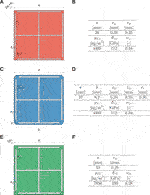FIGURE 2. (A) unit cell giving rise to the metamaterial 1, or in short MM1. (B) Table geometry and material properties of the unit cell: $ρTi$, $ETi$, and $νTi$ stand for the density, Young modulus and Poisson’s ratio of titanium, respectively. (C) unit cell giving rise to the metamaterial 2, or in short MM2. (D) Table geometry and material properties of the unit cell: $ρTi$, $ETi$, and $νTi$ stand for the density, Young modulus and Poisson’s ratio of titanium, respectively. (E) unit cell giving rise to the metamaterial 3, or in short MM3. (F) Table geometry and material properties of the unit cell: $ρSt$, $ESt$, and $νSt$ stand for the density, Young modulus and Poisson’s ratio of steel, respectively.

FIGURE 3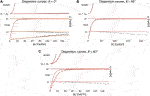FIGURE 3. Dispersion curves for the metamaterial MM1 corresponding to three different direction of propagation (A)$θ=0∘$, (B)$θ=45∘$, and (C)$θ=60∘$.

FIGURE 4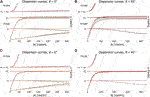FIGURE 4. Dispersion curves for the metamaterial MM2(A, B) and MM3(C, D) corresponding to two direction of propagation (A, C)$θ=0∘$ and (B, D)$θ=45∘$.

All the simulations that are reported in this section and in the next one have been carried on with the software Mathematica for what concerns the relaxed micromorphic semi-analytical solutions, and with the software Comsol for the detailed discrete numerical solutions (see (Aivaliotis et al., 2020) for more details).

### 4.1 Dispersion Curves and Calibration of the Relaxed Micromorphic Elastic Parameters

In this section we provide the relaxed-micromorphic characterization of the three metamaterials previously introduced (Figure 2) by means of the fitting procedure developed in (d’Agostino et al., 2020; Neff et al., 2020). This fitting procedure is based on two different steps aimed at separately characterizing the metamaterial on the static and dynamic regime (d’Agostino et al., 2020; Neff et al., 2020). In particular, the static parameters are identified by remarking that the relaxed micromorphic model tends to a macroscopic equivalent Cauchy medium of stiffness $λmacro$, $μmacro$, and $μmacro*$, when considering the long-wave limit (small frequencies and small wave numbers). These macroscopic parameters can be identified by classical numerical homogenization. They are obtained by imposing periodic boundary conditions that mimic an infinitely extended structure (d’Agostino et al., 2020; Neff et al., 2020). On the other hand, the micro-parameters $λmacro$, $μmacro$, and $μmacro*$ are identified by imposing kinematic uniform boundary conditions on different but equivalent and symmetry-preserving unit cells (Neff et al., 2020). It is important to underline that the micro-parameters so identified estimate is just a lower bound. The parameters $λe$, $μe$, $μe*$ are uniquely identified as a combination of the macro- and micro-elastic parameters thanks to the homogenization formulas derived in (d’Agostino et al., 2020; Neff et al., 2020):

As for the dynamic parameters, four of them can be computed by considering the limiting case $k→0$ (vanishing wavenumbers). In particular, the parameters $L1$, $L2$, $L3$, and $L1*$ can be determined by imposing the cut-off of the relaxed micromorphic model to be equal to the corresponding numerical values obtained, e.g., by Bloch-Floquet analysis. Indeed, the cut-offs frequencies of the relaxed micromorphic model are found to be given by (d’Agostino et al., 2020):

The remaining dynamic parameters $L¯1$, $L¯2$, $L¯3$, and $L¯1*$ are found to have a strong effect on the dispersion curves when $k→∞$ and they are determined by inverse approach to reach the best possible fitting of the Bloch-Floquet dispersion curves (d’Agostino et al., 2020; Aivaliotis et al., 2020).

##### 4.1.1 Relaxed Micromorphic Characterization of the Metamaterial MM1

As a result of the fitting procedure briefly summarized before, the metamaterial MM1 (see Figure 2A) results to be characterized via the relaxed micromorphic parameters give in Table 1A. Table 1B provides the corresponding values of the Cauchy medium obtained as the long wave limit of the relaxed micromorphic medium of Table 1A.

TABLE 1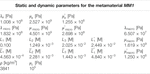TABLE 1. Static and dynamic parameters for the metamaterial MM1. Panel (A) shows the values of the relaxed micromorphic static and dynamic parameters for the metamaterial MM1 determined via the fitting procedure given in (d’Agostino et al., 2020; Aivaliotis et al., 2020). The apparent density ρ is computed based on the titanium microstructure of Figure 2A. Panel (B) shows the values of the equivalent Cauchy continuum elastic coefficients corresponding to the long-wave limit of MM1 computed with the procedure explained in (Neff et al., 2020).

Figure 3 shows the comparison of the dispersion curves obtained via the relaxed micromorphic model with those issued via Bloch-Floquet analysis, for three different directions of propagation.

##### 4.1.2 Relaxed Micromorphic Characterization of the Metamaterials MM2 and MM3

Following the aforementioned procedure, the metamaterials MM2 and MM3 are characterized through the relaxed micromorphic parameters given in Table 2 and Table 3, respectively. The resulting fitting of the dispersion curves is shown in Figure 4.

TABLE 2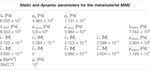TABLE 2. Static and dynamic parameters for the metamaterial MM2. Panel (A) shows the values of the relaxed micromorphic static and dynamic parameters for the metamaterial MM2 determined via the fitting procedures given in (d’Agostino et al., 2020; Aivaliotis et al., 2020). The apparent density ρ is computed based on the titanium microstructure of Figure 2C. Panel (B) shows the values of the equivalent Cauchy continuum elastic coefficients corresponding to the long-wave limit of MM2 computed with the procedure explained in (Neff et al., 2020).

TABLE 3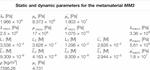TABLE 3. Static and dynamic parameters for the metamaterial MM3. Panel (A) shows the values of the relaxed micromorphic static and dynamic parameters for the metamaterial MM3 determined via the fitting procedures given in (d’Agostino et al., 2020; Aivaliotis et al., 2020). The apparent density ρ is computed based on the steel microstructure of Figure 2E. Panel (B) shows the values of the equivalent Cauchy continuum elastic coefficients corresponding to the long-wave limit of MM3 computed with the procedure explained in (Neff et al., 2020).

## 5 Metastructure’s Refractive Behavior

In this section we will show how the relaxed micromorphic model can be suitably used to describe the reflective properties of a metamaterial’s slab embedded in a homogeneous material (see Figure 5). In what follows, we will restrict ourselves to the microstructure defined in Figure 2A, since the results for the other microstructures are analogous.

FIGURE 5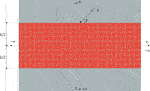FIGURE 5. Schematic representation of a wave with wavenumber k hitting at angle θ a microstructured material slab of thickness h embedded between two isotropic Cauchy media.

We will start by considering the simpler case in which the external homogeneous material is the same as the one used for the metamaterial MM1 (see Figure 2A for its elastic characteristics) and we will then explore how the reflective metamaterial’s behavior changes when changing the outer Cauchy material properties. As we will see, this will allow us to explore the effect of the wavelength of the incident wave on the performances of the relaxed micromorphic model. We will then study how the meta-structure’s behavior as well as the performances of the relaxed micromorphic model vary when increasing the number of unit cells in the metamaterial’s slab.

### 5.1 Dependence of the Metastructure’s Reflective Behavior on the Elastic Properties of the Outer Cauchy Materials

We will show in this subsection that the refractive behavior of the used metamaterial can be drastically modified by simply changing the elastic properties of the outer Cauchy materials. More precisely, the simple fact of changing the properties of the external material can actually “reverse” the metamaterial slab’s refractive behavior (from total reflection to total transmission and vice-versa). This drastic change can be engineered for an extended range of frequencies and angles of incidence. In order to drive the exploration of the more performing structures, we took advantage of the computational performance of the relaxed micromorphic model that allowed us to test different structures in an otherwise unreachable limited time. We show in Figure 6 the dispersion curves of the internal metamaterial, as compared to those of three different “outer” Cauchy materials.

FIGURE 6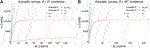FIGURE 6. Acoustic curves of the metamaterial MM1 (continuous lines) as compared to the dispersion curves of three different Cauchy materials (dotted lines). It is highlighted that two dispersion curves coincide since $cp2=cp3$, while a third one is almost superimposed since its value $cs2$ is close to $cp2$ and $cp3$ (see Tables 57 for numerical values of these speeds).

We can see from Figure 6 that, in all considered cases, the external Cauchy material is relatively stiffer than the internal metamaterial in the long-wave limit, especially with the reference to the shear modulus. This can be inferred by recalling that the slope of the acoustic curves at the origin represents the speed of propagation ($cp$ and $cs$) of the corresponding waves (see Table 4) and that these speeds are directly related to the metamaterial’s elastic properties in the long wave limit (see Table 1).

TABLE 4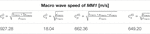TABLE 4. Wave speed expressions for the Cauchy material which is the long-wave limit of MM1. The expressions for the pressure and shear waves are explicitly given for the two directions of propagation $θ=0$ and $θ=π/4$. Such speeds are the slopes of the tangents at the origin of the acoustic dispersion curves in Figures 3A,B and are computed based on the macro parameters given in the table in Figure 2A.

TABLE 5
TABLE 6
TABLE 7

The three outer Cauchy materials have been chosen starting from the same material as the one constituting the metamaterial MM1 (titanium) and then lowering the propagation speeds $cp$ and $cs$ so as to widen the range of frequencies for which the relaxed micromorphic model gives quantitatively good results.7 Indeed, with reference to Figures 79, we can remark that the frequency interval for which the relaxed micromorphic model is predictive of the microstructure’s reflective behavior is larger for the “softer” outer Cauchy material CM3 (Figure 9). Indeed, the fact of considering a “softer” outer material is equivalent to say that, at any given frequency, the corresponding wavelength of the traveling incident wave is smaller than the one of the wave propagating in the “stiffer” material. It is known that, as far as a homogenized model is concerned, its accuracy for the study of a problem of the type presented in this paper, may depend on three different characteristic lengths, namely:

the wavelength of the traveling incident wave;

the thickness of the metamaterials’s slab;

the characteristic size of the unit cell.

FIGURE 7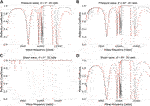FIGURE 7. Comparison of the microstructure’s (black line) and micromorphic (red line) reflection coefficient as a function of frequency for a 20 unit cells slab of MM1 and embedded in CM1. (A) “pressure” normal incident wave with respect to the slab’s interface. (B) “pressure” 45° incident wave with respect to the slab’s interface. (C) “shear” incident wave normal to the slab’s interface. (D) “shear” 45° incident wave with respect to the slab’s interface.

Having fixed the unit cell’s dimensions for applications in acoustic control, we do not discuss here the influence of the third characteristic length. As for the influence of the thickness of the metamaterial’s slab, we refer to Section 5.2.

Here, we limit ourselves to remark that, given the intrinsic simplifications associated to a continuum model, a threshold value $ℓt$ for the wavelength of the incident wave exists, below which the model starts loosing its predictive capabilities. For the meta-structures of Figures 7, 8 this threshold value is reached already for frequencies slightly higher than the metamaterial’s band-gap. When considering the “softer” outer Cauchy material CM3, the wavelength of the incident wave remains lower than the threshold $ℓt$ for a larger frequency range that exceeds the band-gap (see Figure 9). Being aware of the existence of such threshold $ℓt$ is essential for a correct use of enriched continuum models over the appropriate frequency ranges.

FIGURE 8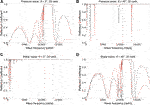FIGURE 8. Comparison of the microstructure’s (black line) and micromorphic (red line) reflection coefficient as a function of frequency for a 20 unit cells slab of MM1 and embedded in CM2. (A) “pressure” normal incident wave with respect to the slab’s interface. (B) “pressure” 45° incident wave with respect to the slab’s interface. (C) “shear” incident wave normal to the slab’s interface. (D) “shear” 45° incident wave with respect to the slab’s interface.

FIGURE 9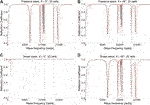FIGURE 9. Comparison of the microstructure’s (black line) and micromorphic (red line) reflection coefficient as a function of frequency for a 20 unit cells slab of MM1 and embedded in CM3. (A) “pressure” normal incident wave with respect to the slab’s interface. (B) “pressure” 45° incident wave with respect to the slab’s interface. (C) “shear” incident wave normal to the slab’s interface. (D) “shear” 45° incident wave with respect to the slab’s interface.

### 5.2 Dependence of the Metamaterial’s Reflective Behavior on the Thickness of the Slab

In this subsection, we show to which extent the reflective behavior of the metamaterial’s slab is influenced by the thickness of the slab itself. At the same time, we are able to show that the performances of the relaxed micromorphic model increases when increasing the thickness of the slab.

Indeed, by comparison of Figure 10 with Figure 7, it is possible to infer that the fact of increasing the slab’s thickness mainly acts on the number and amplitude of oscillations that occur in the reflection coefficient for frequencies lower and higher than the band-gap. Moreover, it can be seen from these figures that the performances of the relaxed micromorphic model is improved when increasing the number of unit cells constituting the metamaterial slab embedded in the Cauchy material CM1. Nevertheless, some mismatch can still be observed for frequencies higher than the band-gap, also for relatively high number of unit cells. This high-frequency mismatch is related to the fact that the wavelength of the incident wave exceeds the threshold value $ℓt$ as discussed before in sub Section 5.1.

FIGURE 10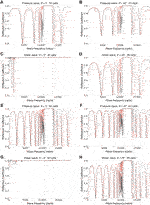FIGURE 10. Comparison of the microstructure’s (dashed black line) and micromorphic (red line) reflection coefficient as a function of frequency for a 30 (A–D) and a 60 (E–H) unit cells slab of MM1 and embedded in CM1. (A) and (E) “pressure” normal incident wave with respect to the slab’s interface for a 25 and 30 cells respectively. (B) and (F) “pressure” 45° incident wave with respect to the slab’s interface for a 25 and 30 cells, respectively. (C) and (G) “shear” incident wave normal to the slab’s interface for a 25 and 30 cells, respectively. (D) and (H) “shear” 45° incident wave with respect to the slab’s interface for a 25 and 30 cell,s respectively.

To improve the higher-frequency micromorphic description of the structure in this case, a substantial constitutive extension of the relaxed micromorphic model is needed so as to account for higher frequency modes that presumably play an important role in this frequency range. Similar arguments are valid for the slab embedded in the Cauchy material CM2, as shown by Figure 11 and Figure 8. As for the slab embedded in the Cauchy material CM3, we already observed in Figure 9 that its reflective behavior is better caught by the relaxed micromorphic model than in the previous case, even at higher frequency.

FIGURE 11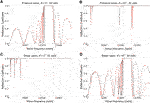FIGURE 11. Comparison of the microstructure’s (black line) and micromorphic (red line) reflection coefficient as a function of frequency for a 30 unit cells slab of MM1 and embedded in CM2. (A) “pressure” normal incident wave with respect to the slab’s interface. (B) “pressure” 45° incident wave with respect to the slab’s interface. (C) “shear” incident wave normal to the slab’s interface. (D) “shear” 45° incident wave with respect to the slab’s interface.

This means that, for this structure, the relaxed micromorphic model presented is sufficient for its correct description in the considered frequency range and that its generalization can be avoided in this case, also for relatively high frequencies. Indeed, in this last case, the fact of increasing the number of unit cells does not significantly improve the description of the slab’s refractive behavior (see Figure 12). The slight differences between the reflection patterns obtained via the relaxed micromorphic model and those obtained via the full simulations (see Figure 12) can hence be attributed uniquely to a constitutive enhancement of the relaxed micromorphic model to include extra degrees of freedom and higher modes.

FIGURE 12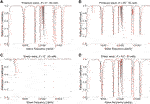FIGURE 12. Comparison of the microstructure’s (black line) and micromorphic (red line) reflection coefficient as a function of frequency for a 30 unit cells slab of MM1 and embedded in CM3. (A) and (E) “pressure” normal incident wave with respect to the slab’s interface for a 25 and 30 cells respectively. (B) and (F) “pressure” 45° incident wave with respect to the slab’s interface for a 25 and 30 cells, respectively. (C) and (G) “shear” incident wave normal to the slab’s interface for a 25 and 30 cells, respectively. (D) and (H) “shear” 45° incident wave with respect to the slab’s interface for a 25 and 30 cells, respectively.

### 5.3 Reflection Coefficient of the Tetragonal Metamaterial Slab as a Function of the Angle of Incidence θ and of the Wave-Frequency

Since the predictive capability of the relaxed micromorphic model here proposed has been assessed in the previous sections, in this subsection, we show the behavior of the reflection coefficient as a function of the frequency of the incident wave and the angle of incidence for the structure of Figure 2A with outer Cauchy material CM1.

We start noticing that for the case of an incident “pressure” wave the structure’s refractive behavior is relatively unaffected by the value of the angle of incidence (see Figures 13A,C).

FIGURE 13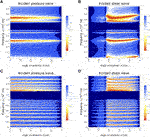FIGURE 13. Simulation of the reflection coefficient with the obtained relaxed micromorphic model for a 20 (A, B) and a 100 (C, D) unit cells thick slab made up of MM1 material and embedded in CM1 Cauchy as function of the angle of incidence and of the wave-frequency–(A) and (C): incident pressure wave; (B) and (D) incident shear wave.

In particular, when considering fewer unit cells in the metamaterial slab (Figure 13A), we can observe almost total reflection occurring in a wide frequency range (extending outside the band-gap) and for all angles of incidence. Few frequencies can be identified around which total transmission occurs. When increasing the number of cells in the metamaterial slab, the frequencies around which total transmission occurs increase in number. We can thus remark that the simple fact of considering a finite-size metamaterial with a different number of unit cells modifies the structure’s behavior in a significant way.

Things are even more interesting when considering “shear” incident waves, since the structure’s behavior starts being significantly affected by the angle of incidence of the traveling wave.

In particular, a critical angle exists (see Figures 13B,D) such that all waves hitting the interface with an angle included between normal incidence and this critical value are almost completely reflected for any frequency (even outside the band-gap). For angles beyond the critical value the structure’s behavior becomes similar to that observed for incident “pressure” waves.

We analyze the same meta-structure of Figure 2A by now considering the material CM2 as “outer” Cauchy material. By direct comparison of Figure 14 with Figure 13, it can be easily inferred that the meta-structure’s behavior is somehow reversed with respect to the previous structure.

FIGURE 14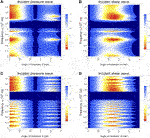FIGURE 14. Simulation of the reflection coefficient with the obtained relaxed micromorphic model for a 20 (A, B) and a 100 (C, D) unit cells thick slab made up of MM1 material and embedded in CM2 Cauchy as function of the angle of incidence and of the wave-frequency–(A) and (C): incident pressure wave; (B) and (D) incident shear wave.

First of all, we can identify a “critical angle region” for incident “pressure” waves instead than for “shear” ones. Moreover, we can remark that an almost total transmission occurs for angles smaller than this critical value instead than a total reflection in the previous case.

For “shear” incident waves the behavior is still different because different zones are identified depending on the value of the incident angle. In particular, two critical angles exist in this case such that total transmission occurs between these two critical values, while total reflection occurs otherwise.

In summary, we can see how the fact of simply changing the properties of the “outer” Cauchy material reverses the meta-structure’s behavior in specific frequency and angle-of-incidence ranges, both for “pressure” and “shear” incident waves.

## 6 Conclusion

In this paper we use the relaxed micromorphic model to characterize three different 2D tetragonal metamaterials that can be used for applications in acoustic control. The reduced structure of the relaxed micromorphic model allows us to efficiently explore different meta-structural configurations in which a metamaterial’s slab is embedded in a homogeneous Cauchy material. As a result, we are able to show that the metamaterial’s refractive behavior can be drastically changed by simply acting on the stiffness of the homogeneous material. In this way, the same structure can be adapted so as to act as a total screen or a total absorber in specific frequency and angle-of-incidence ranges. The results presented so far, clearly show that the study of the mechanical behavior of metamaterials cannot be disjoined by the study of their interactions with other materials, if one wants to enable the realistic conception of new engineering meta-structures. By presenting our results, we also outline that, as any model, also enriched models have limitations that have to be identified “a priori” to avoid their inappropriate use. In particular, we underline that the model’s performances may depend on three characteristic lengths that are related to 1) the unit cell’s size, 2) the metamaterial’s slab thickness and 3) the wavelength of the traveling wave. Depending on the relative proportions of these three characteristic lengths the relaxed micromorphic model will be more or less efficient in the description of the meta-structure’s behavior over an extended frequency range. Indeed, while the relaxed micromorphic model will always be predictive of this behavior in the long wave limit, more or less marked differences may emerge for higher frequencies and lower wavelengths. This calls for the formulation of a new enriched model, including extra degrees of freedom and suitable dynamic internal lengths, so as to extend its efficiency to higher-frequency/smaller-wavelength regions for a wide panel of external excitations.

## Data Availability Statement

The raw data supporting the conclusions of this article will be made available by the authors, without undue reservation.

## Author Contributions

GR has developed and performed all the numerical and semi-analytical simulations presented in the paper, MC, FD, BE, and PN developed the microstructures suitable for acoustic control and characterized them through the Relaxed Micromorphic Model, PN and AM conceived the appropriate structure of the Relaxed Micromorphic Model, AM coordinated the research, established the structure of the paper and designed the cases of interest of the meta-structures under study.

## Conflict of Interest

The authors declare that the research was conducted in the absence of any commercial or financial relationships that could be construed as a potential conflict of interest.

The reviewer MM declared a past co-authorship with several of the authors MC, PN, AM to the handling editor.

## Footnotes

1The relaxed micromorphic model can be used to qualitatively describe the mechanical response of 2D and 3D metamaterials whose class of symmetry can be represented via fourth order elastic tensors. The only class of symmetry that is currently excluded is the hexagonal one, for which the introduction of higher order elastic tensors will be needed.

2For example, $(A⋅v)i=Aijvj$, $(A⋅B)ik=AijBjk$, $(C⋅B)ijk=CijpBpk$, $(C:B)i=CijpBpj$, $〈v,w〉=v⋅w=viwi$, $〈A,B〉=AijBij$, etc.

3The operators $∇$, curl and Div are the classical gradient, curl and divergence operators. In symbols, for a field u of any order, $(∇u)i=u,i$, for a vector field v, $(curlv)i=ϵijkvk,j$ and for a field w of order $k>1$, $(Div w)i1i2…ik−1=wi1i2…ik,ik$.

4Being reserved to the time variable, the index t is treated separately and does not comply with Einstein notation.

5The presence of curvature terms is essential to catch size-effects in the static regime that are not the target of the present paper.

6When performing the calibration of the inertia parameters, we could establish that the characteristic lengths $L1$, $L2$, $L3$, and $L1*$ are related to the high-frequency/large-wavelength metamaterial’s response, in particular they directly intervene in the expressions of the cut-off frequencies. On the other hand, the characteristic lengths $L¯1$, $L¯2$, $L¯3$, and $L¯1*$ have a significant effect on the high-frequency/short-wavelength metamaterial’s response, since they visibly increase dispersive behaviors associated to the asymptotic part of the dispersion curves.

7We lowered the propagation speeds $cp$ and $cs$ by directly changing the values of the stiffness of the outer metamaterial or equivalently by increasing the density as shown in Tables 57 so as to meet these propagation speeds. This means that different materials CM1, CM2, and CM3 can be found that have the same speeds as in Tables 57. All these materials would give rise to the meta-structure’s behaviors presented in this section.

## References

Aivaliotis, A., Daouadji, A., Barbagallo, G., Tallarico, D., Neff, P., and Madeo, A. (2018). Low-and high-frequency stoneley waves, reflection and transmission at a cauchy/relaxed micromorphic interface. Preprint repository name [Preprint]. Available at: arXiv:1810.12578 (Accessed October 30, 2018).

Aivaliotis, A., Daouadji, A., Barbagallo, G., Tallarico, D., Neff, P., and Madeo, A. (2019a). Microstructure-related stoneley waves and their effect on the scattering properties of a 2d cauchy/relaxed-micromorphic interface. Wave Motion 90, 99–120. doi:10.1016/j.wavemoti.2019.04.003

Aivaliotis, A., Tallarico, D., d’Agostino, M., Daouadji, A., Neff, P., and Madeo, A. (2020). Frequency-and angle-dependent scattering of a finite-sized meta-structure via the relaxed micromorphic model. Arch. Appl. Mech. 90, 1–24. doi:10.1007/s00419-019-01651-9

Aivaliotis, A., Tallarico, D., D’Agostino, M., Daouadji, A., Neff, P., and Madeo, A. (2019b). Relaxed micromorphic broadband scattering for finite-size meta-structures–a detailed development. Preprint repository name [Preprint]. Available at: arXiv:1905.12297 (Accessed May 29, 2019).

Allaire, G. (1992). Homogenization and two-scale convergence. SIAM J. Math. Anal. 23, 1482–1518. doi:10.1137/0523084

Andrianov, I., Bolshakov, V., Danishevs’kyy, V., and Weichert, D. (2008). Higher order asymptotic homogenization and wave propagation in periodic composite materials. Proc. Math. Phys. Eng. Sci. 464, 1181–1201. doi:10.1098/rspa.2007.0267

Bacigalupo, A., and Gambarotta, L. (2014). Second-gradient homogenized model for wave propagation in heterogeneous periodic media. Int. J. Solid Struct. 51, 1052–1065. doi:10.1016/j.ijsolstr.2013.12.001

Barchiesi, E., Spagnuolo, M., and Placidi, L. (2019). Mechanical metamaterials: a state of the art. Math. Mech. Solid 24, 212–234. doi:10.1177/1081286517735695

Bensoussan, A., Lions, J., and Papanicolaou, G. (2011). Asymptotic analysis for periodic structures. Amsterdam, Netherlands: American Mathematical Society, 374, 392.

Bertoldi, K., Vitelli, V., Christensen, J., and van Hecke, M. (2017). Flexible mechanical metamaterials. Nat. Rev. Mater. 2, 1–11. doi:10.1038/natrevmats.2017.66

Bigoni, D., and Movchan, A. (2002). Statics and dynamics of structural interfaces in elasticity. Int. J. Solid Struct. 39, 4843–4865. doi:10.1016/s0020-7683(02)00416-x

Bilal, O., Ballagi, D., and Daraio, C. (2018). Architected lattices for simultaneous broadband attenuation of airborne sound and mechanical vibrations in all directions. Phys. Rev. Appl. 10, 054060. doi:10.1103/physrevapplied.10.054060

Bilal, O., Süsstrunk, R., Daraio, C., and Huber, S. (2017). Intrinsically polar elastic metamaterials. Adv. Mater. 29, 1700540. doi:10.1002/adma.201700540

Bordiga, G., Cabras, L., Piccolroaz, A., and Bigoni, D. (2019). Prestress tuning of negative refraction and wave channeling from flexural sources. Appl. Phys. Lett. 114, 041901. doi:10.1063/1.5084258

Bouchitté, G., and Bellieud, M. (2002). Homogenization of a soft elastic material reinforced by fibers. Asymptot. Anal. 32, 153–183.

Boutin, C., Rallu, A., and Hans, S. (2014). Large scale modulation of high frequency waves in periodic elastic composites. J. Mech. Phys. Solid 70, 362–381. doi:10.1016/j.jmps.2014.05.015

Brun, M., Guenneau, S., Movchan, A., and Bigoni, D. (2010). Dynamics of structural interfaces: filtering and focussing effects for elastic waves. J. Mech. Phys. Solid 58, 1212–1224. doi:10.1016/j.jmps.2010.06.008

Bückmann, T., Kadic, M., Schittny, R., and Wegener, M. (2015). Mechanical cloak design by direct lattice transformation. Proc. Natl. Acad. Sci. Unit. States Am. 112, 4930–4934. doi:10.1073/pnas.1501240112

Camar-Eddine, M., and Seppecher, P. (2003). Determination of the closure of the set of elasticity functionals. Arch. Ration. Mech. Anal. 170, 211–245. doi:10.1007/s00205-003-0272-7

Celli, P., Yousefzadeh, B., Daraio, C., and Gonella, S. (2019). Bandgap widening by disorder in rainbow metamaterials. Appl. Phys. Lett. 114, 091903. doi:10.1063/1.5081916

Chen, W., and Fish, J. (2001). A dispersive model for wave propagation in periodic heterogeneous media based on homogenization with multiple spatial and temporal scales. J. Appl. Mech. 68, 153–161. doi:10.1115/1.1357165

Coulais, C., Teomy, E., De Reus, K., Shokef, Y., and Van Hecke, M. (2016). Combinatorial design of textured mechanical metamaterials. Nature 535, 529–532. doi:10.1038/nature18960

Craster, R., Kaplunov, J., and Pichugin, A. (2010). High-frequency homogenization for periodic media. Proc. Math. Phys. Eng. Sci. 466, 2341–2362. doi:10.1098/rspa.2009.0612

Cummer, S., Christensen, J., and Alù, A. (2016). Controlling sound with acoustic metamaterials. Nat. Rev. Mater. 1, 16001. doi:10.1038/natrevmats.2016.1

Deymier, P. (2013). Acoustic metamaterials and phononic crystals Berlin, Heidelberg: Springer Science and Business Media, 173, 379.

d’Agostino, M., Barbagallo, G., Ghiba, I., Eidel, B., Neff, P., and Madeo, A. (2020). Effective description of anisotropic wave dispersion in mechanical band-gap metamaterials via the relaxed micromorphic model. J. Elasticity. 39, 299–329. doi:10.1007/s10659-019-09753-9

El Sherbiny, M., and Placidi, L. (2018). Discrete and continuous aspects of some metamaterial elastic structures with band gaps. Arch. Appl. Mech. 88, 1725–1742. doi:10.1007/s00419-018-1399-1

Geers, M., Kouznetsova, V., and Brekelmans, M. (2010). Multi-scale computational homogenization: trends and challenges. J. Comput. Appl. Math. 234, 2175–2182. doi:10.1016/j.cam.2009.08.077

Goh, H., and Kallivokas, L. (2019). Inverse metamaterial design for controlling band gaps in scalar wave problems. Wave Motion 88, 85–105. doi:10.1016/j.wavemoti.2019.02.001

Guenneau, S., Movchan, A., Pétursson, G., and Ramakrishna, A. (2007). Acoustic metamaterials for sound focusing and confinement. New J. Phys. 9, 399. doi:10.1088/1367-2630/9/11/399

Hashin, Z., and Shtrikman, S. (1963). A variational approach to the theory of the elastic behaviour of multiphase materials. J. Mech. Phys. Solid 11, 127–140. doi:10.1016/0022-5096(63)90060-7

Hill, R. (1963). Elastic properties of reinforced solids: some theoretical principles. J. Mech. Phys. Solid 11, 357–372. doi:10.1016/0022-5096(63)90036-x

Hu, R., and Oskay, C. (2017). Nonlocal homogenization model for wave dispersion and attenuation in elastic and viscoelastic periodic layered media. J. Appl. Mech. 84, 031003. doi:10.1115/1.4035364

Hussein, M., Leamy, M., and Ruzzene, M. (2014). Dynamics of phononic materials and structures: historical origins, recent progress, and future outlook. Appl. Mech. Rev. 66, 040802. doi:10.1115/1.4026911

Kadic, M., Bückmann, T., Schittny, R., Gumbsch, P., and Wegener, M. (2014). Pentamode metamaterials with independently tailored bulk modulus and mass density. Phys. Rev. Appl. 2, 054007. doi:10.1103/physrevapplied.2.054007

Kaina, N., Causier, A., Bourlier, Y., Fink, M., Berthelot, T., and Lerosey, G. (2017). Slow waves in locally resonant metamaterials line defect waveguides. Sci. Rep. 7, 1–11. doi:10.1038/s41598-017-15403-8

Kochmann, D., and Bertoldi, K. (2017). Exploiting microstructural instabilities in solids and structures: from metamaterials to structural transitions. Appl. Mech. Rev. 69, 050801. doi:10.1115/1.4037966

Koutsianitis, P., Tairidis, G., Drosopoulos, G., and Stavroulakis, G. (2019). Conventional and star-shaped auxetic materials for the creation of band gaps. Arch. Appl. Mech. 89, 2545–2562. doi:10.1007/s00419-019-01594-1

Krushynska, A., Miniaci, M., Bosia, F., and Pugno, N. (2017). Coupling local resonance with Bragg band gaps in single-phase mechanical metamaterials. Ext. Mech. Lett. 12, 30–36. doi:10.1016/j.eml.2016.10.004

Lakes, R. (1987). Foam structures with a negative Poisson’s ratio. Science 235, 1038–1041. doi:10.1126/science.235.4792.1038

Liu, Z., Zhang, X., Mao, Y., Zhu, Y., Yang, Z., Chan, C., et al. (2000). Locally resonant sonic materials. Science 289, 1734–1736. doi:10.1126/science.289.5485.1734

Lustig, B., Elbaz, G., Muhafra, A., and Shmuel, G. (2019). Anomalous energy transport in laminates with exceptional points. J. Mech. Phys. Solid 133, 103719. doi:10.1016/j.jmps.2019.103719

Madeo, A., Barbagallo, G., Collet, M., D’agostino, M., Miniaci, M., and Neff, P. (2018a). Relaxed micromorphic modeling of the interface between a homogeneous solid and a band-gap metamaterial: new perspectives towards metastructural design. Math. Mech. Solid 23, 1485–1506. doi:10.1177/1081286517728423

Madeo, A., Collet, M., Miniaci, M., Billon, K., Ouisse, M., and Neff, P. (2018b). Modeling phononic crystals via the weighted relaxed micromorphic model with free and gradient micro-inertia. J. Elasticity 130, 59–83. doi:10.1007/s10659-017-9633-6

Madeo, A., Neff, P., Ghiba, I., and Rosi, G. (2016). Reflection and transmission of elastic waves in non-local band-gap metamaterials: a comprehensive study via the relaxed micromorphic model. J. Mech. Phys. Solid 95, 441–479. doi:10.1016/j.jmps.2016.05.003

Miehe, C., Schröder, J., and Schotte, J. (1999). Computational homogenization analysis in finite plasticity simulation of texture development in polycrystalline materials. Comput. Methods Appl. Mech. Eng. 171, 387–418. doi:10.1016/s0045-7825(98)00218-7

Milton, G. (2002). “The theory of composites,” in Cambridge monographs on applied and computational mathematics Cambridge, United Kingdom: University of Cambridge, 719.

Milton, G., and Cherkaev, A. (1995). Which elasticity tensors are realizable. J. Eng. Mater. Technol. 117, 483–493. doi:10.1115/1.2804743

Miniaci, M., Pal, R., Manna, R., and Ruzzene, M. (2019). Valley-based splitting of topologically protected helical waves in elastic plates. Phys. Rev. B. 100, 024304. doi:10.1103/physrevb.100.024304

Misseroni, D., Colquitt, D., Movchan, A., Movchan, N., and Jones, I. (2016). Cymatics for the cloaking of flexural vibrations in a structured plate. Sci. Rep. 6, 23929. doi:10.1038/srep23929

Misseroni, D., Movchan, A., and Bigoni, D. (2019). Omnidirectional flexural invisibility of multiple interacting voids in vibrating elastic plates. Proc. Royal Soc. A. 475, 20190283. doi:10.1098/rspa.2019.0283

Morini, L., Eyzat, Y., and Gei, M. (2019). Negative refraction in quasicrystalline multilayered metamaterials. J. Mech. Phys. Solid 124, 282–298. doi:10.1016/j.jmps.2018.10.016

Neff, P., Eidel, B., d’Agostino, M., and Madeo, A. (2020). Identification of scale-independent material parameters in the relaxed micromorphic model through model-adapted first order homogenization. J. Elasticity. 139, 269–298. doi:10.1007/s10659-019-09752-w

Norris, A., Amirkulova, F., and Parnell, W. (2014). Active elastodynamic cloaking. Math. Mech. Solid 19, 603–625. doi:10.1177/1081286513479962

Park, C., and Lee, S. (2019). Zero-reflection acoustic metamaterial with a negative refractive index. Sci. Rep. 9, 1–7. doi:10.1038/s41598-019-40184-7

Pideri, C., and Seppecher, P. (1997). A second gradient material resulting from the homogenization of an heterogeneous linear elastic medium. Continuum Mech. Therm. 9, 241–257. doi:10.1007/s001610050069

Romano, G., Barretta, R., and Diaco, M. (2016). Micromorphic continua: non-redundant formulations. Continuum Mech. Therm. 28, 1659–1670. doi:10.1007/s00161-016-0502-5

Sánchez-Palencia, E. (1980). Non-homogeneous media and vibration theory. Lect. Notes Phys. 127, 400. doi:10.1007/3-540-10000-8

Sridhar, A., Kouznetsova, V., and Geers, M. (2018). A general multiscale framework for the emergent effective elastodynamics of metamaterials. J. Mech. Phys. Solid 111, 414–433. doi:10.1016/j.jmps.2017.11.017

Sridhar, A., Kouznetsova, V., and Geers, M. (2016). Homogenization of locally resonant acoustic metamaterials towards an emergent enriched continuum. Comput. Mech. 57, 423–435. doi:10.1007/s00466-015-1254-y

Srivastava, A. (2016). Metamaterial properties of periodic laminates. J. Mech. Phys. Solid 96, 252–263. doi:10.1016/j.jmps.2016.07.018

Srivastava, A., and Nemat-Nasser, S. (2014). On the limit and applicability of dynamic homogenization. Wave Motion. 51, 1045–1054. doi:10.1016/j.wavemoti.2014.04.003

Srivastava, A., and Willis, J. (2017). Evanescent wave boundary layers in metamaterials and sidestepping them through a variational approach. Proc. Math. Phys. Eng. Sci. 473, 20160765. doi:10.1098/rspa.2016.0765

Suquet, P. (1985). Elements of homogenization for inelastic solid Mechanics, homogenization techniques for composite media. Lect. Notes Phys. 272, 193. doi:10.1007/3-540-17616-0

Tallarico, D., Trevisan, A., Movchan, N., and Movchan, A. (2017). Edge waves and localization in lattices containing tilted resonators. Front. Mater. 4, 16. doi:10.3389/fmats.2017.00016

Wang, P., Casadei, F., Shan, S., Weaver, J., and Bertoldi, K. (2014). Harnessing buckling to design tunable locally resonant acoustic metamaterials. Phys. Rev. Lett. 113, 014301. doi:10.1103/PhysRevLett.113.014301

Wang, Y., Wang, T., Liang, J., Wang, Y., and Laude, V. (2018). Channeled spectrum in the transmission of phononic crystal waveguides. J. Sound Vib. 437, 410–421. doi:10.1016/j.jsv.2018.09.030

Willis, J. (1977). Bounds and self-consistent estimates for the overall properties of anisotropic composites. J. Mech. Phys. Solid 25, 185–202. doi:10.1016/0022-5096(77)90022-9

Willis, J. (2011). Effective constitutive relations for waves in composites and metamaterials. Proc. Math. Phys. Eng. Sci. 467, 1865–1879. doi:10.1016/j.mechmat.2009.01.010

Willis, J. (2009). Exact effective relations for dynamics of a laminated body. Mech. Mater. 41, 385–393. doi:10.1098/rspa.2010.0620

Willis, J. (2016). Negative refraction in a laminate. J. Mech. Phys. Solid 97, 10–18. doi:10.1016/j.crme.2012.02.001

Willis, J. (2012). The construction of effective relations for waves in a composite. Compt. Rendus Mec. 340, 181–192. doi:10.1016/j.jmps.2015.11.004

Zhao, W., Yang, Y., Tao, Z., and Hang, Z. (2018). Tunable transmission and deterministic interface states in double-zero-index acoustic metamaterials. Sci. Rep. 8, 1–9. doi:10.1038/s41598-018-24773-6

Zhou, L., and Kriegsmann, G. (2007). Complete transmission through a periodically perforated rigid slab. J. Acoust. Soc. Am. 121, 3288–3299. doi:10.1121/1.2721878

Zhu, R., Liu, X., and Huang, G. (2015). Study of anomalous wave propagation and reflection in semi-infinite elastic metamaterials. Wave Motion. 55, 73–83. doi:10.1016/j.wavemoti.2014.12.007

Keywords: mechanical metamaterials, wave-propagation, meta-materials, meta-structure, relaxed micromorphic model

Citation: Rizzi G, Collet M, Demore F, Eidel B, Neff P and Madeo A (2021) Exploring Metamaterials’ Structures Through the Relaxed Micromorphic Model: Switching an Acoustic Screen Into an Acoustic Absorber. Front. Mater. 7:589701. doi: 10.3389/fmats.2020.589701

Received: 31 July 2020; Accepted: 23 September 2020;
Published: 17 March 2021.

Edited by:

Diego Misseroni, University of Trento, Italy

Reviewed by:

Marco Miniaci, Swiss Federal Laboratories for Materials Science and Technology, Switzerland
Lorenzo Morini, Cardiff University, United Kingdom

Copyright © 2021 Rizzi, Collet, Demore, Eidel, Neff and Madeo. This is an open-access article distributed under the terms of the Creative Commons Attribution License (CC BY). The use, distribution or reproduction in other forums is permitted, provided the original author(s) and the copyright owner(s) are credited and that the original publication in this journal is cited, in accordance with accepted academic practice. No use, distribution or reproduction is permitted which does not comply with these terms.

*Correspondence: Patrizio Neff, patrizio.neff@uni-due.de